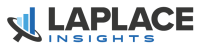# Standard Deviation as a Measure of RiskThere are many ways to measure and assess risk in an investment.  The standard deviation is one such way and it measures how much returns change over a period.  This is also known as the volatility of returns.

Conceptually, the standard deviation measures the typical deviation from the mean return.  The mean return is the average return of the asset over a specified period.

For example, consider the following set of monthly returns calculated from the  closing price on the popular SPDR ETF tracking the S&P 500 (SPY), during 2011:

The mean (or average) monthly return during 2011 is very close to zero, at 0.08%.  If you only look at that number, you would think this was a rather quiet year.  What’s more, the average return is NOT the same as the return obtained during the period.  The price at the end of 2010 was \$125.75, while it was \$125.50 at the end of 2011.  So excluding dividends, you actually lost a tiny bit of money during the year.## Reality Hits with the Standard Deviation

Looking at either the price endpoints or the average monthly returns, we have the impression that 2011 was one of the most boring year in the stock market ever recorded.  However, the reality couldn’t be more different.  This was the year of the US budget crisis, when Congress was threatening to shut down the government over disagreement on the budget.  Such great political wisdom sent the stock market in a series of highly volatile and uncertain months.

This is where the standard deviation gives us a more complete story of what really happened to the monthly returns.  At 4.5%, it tells us that the “typical” month returns was about 4.5%, either up or down.  Many months had much smaller returns.  For instance, March, November and December were essentially flat at -0.4%, +0.4% and +0.4% respectively.  On the other hand, the summer was not exactly a beach vacation:  August, September and October posted returns of -5.5%, -7.4% and +10.9% respectively.  The standard deviation measures a sort of average absolute movement and it is always expressed as a positive number.  This is because it tells us about the typical return magnitude (or change in price), without making any statement about its direction.  Remember the objective is to measure volatility, not price direction.

In mathematical form, the standard deviation is defined as the square root of the variance.  The variance is the average of the squared difference from the mean.  Mathematically, this is expressed as follows:Consider having a look at the Wikipedia definition for more on variance and standard deviation. The standard deviation is a very useful measure of risk when evaluating investment strategies.